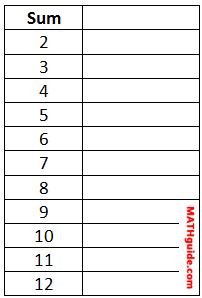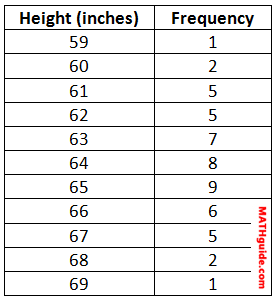Frequency Tables
 Home > Lessons > Frequency Tables Search | Updated March 21st, 2018
Introduction

This lesson page will inform you how to calculate the limit of a function for extreme values. Here are the sections within this lesson page:

 Introduction People collect data of all kinds. At a doctor's office, heights, weights, blood pressures, and pulse rates are routinely collected. Quality control workers test items to see if they are ready to leave a factory or to determine if production is building materials as they should be made. Teachers, school administrators, and state officials study trends in test data to see if a school is performing according to standards.     This page will inform you how to organize data into frequency tables.
 Creating Frequency Tables Imagine rolling a pair of 6-sided dice and you recorded the sum of the two dice. Each die that is rolled can produce a number 1 through 6. So, the sum of the dice would be anywhere from 2 to 12.     Let us say we rolled those dice and recorded the sums. Here could be that list of sums.We could place these sum in order, from smallest to largest.We could also place these numbers in a table. Since rolling dice and adding them gives us outcomes anywhere from 2 - 12, we can place those possible sums in one column of a table, like so.In the next column, we can place how many times those sums occur. For instance, there are two 2s and four 7s. We can place those values in the table for all sums, like so.The second column is called the frequency. Placing that title at the top completes our frequency table, which has been placed below.uiz: Frequency Tables Reading Frequency Tables If a healthcare worker at a local high school measured the heights of a group of freshmen and tallied them, this could be the result.We could take the data from this table and report it instead as a data set. We would have to write one 59, two 60s, five 61s, and so on, like so.uiz: Frequency Tables Interactive Quizmasters Try this interactive quizmaster to determine if you understand frequency tables.uiz: Frequency Tables Related Lessons and Quizzes Try this related material.esson: Standard Deviationesson: Bell Curve: 68-95-99 Ruleesson: Margin of Error and Confidence Intervalsesson: Margin of Error & Confidence Intervals by Formulauiz: Dice Roller: Mean, Standard Deviation